# 7.3 Unit circle  (Page 8/11)

 Page 8 / 11

$\mathrm{sin}\text{\hspace{0.17em}}0$

## Numeric

For the following exercises, state the reference angle for the given angle.

$240°$

$60°$

$-170°$

$100°$

$80°$

$-315°$

$135°$

$45°$

$\frac{5\pi }{4}$

$\frac{2\pi }{3}$

$\frac{\pi }{3}$

$\frac{5\pi }{6}$

$\frac{-11\pi }{3}$

$\frac{\pi }{3}$

$\frac{-7\pi }{4}$

$\frac{-\pi }{8}$

$\frac{\pi }{8}$

For the following exercises, find the reference angle, the quadrant of the terminal side, and the sine and cosine of each angle. If the angle is not one of the angles on the unit circle, use a calculator and round to three decimal places.

$225°$

$300°$

$60°,$ Quadrant IV, $\text{\hspace{0.17em}}\text{sin}\left(300°\right)=-\frac{\sqrt{3}}{2},\mathrm{cos}\left(300°\right)=\frac{1}{2}$

$320°$

$135°$

$45°,$ Quadrant II, $\text{sin}\left(135°\right)=\frac{\sqrt{2}}{2},\mathrm{cos}\left(135°\right)=-\frac{\sqrt{2}}{2}$

$210°$

$120°$

Quadrant II, $\text{\hspace{0.17em}}\text{sin}\left(120°\right)=\frac{\sqrt{3}}{2},\mathrm{cos}\left(120°\right)=-\frac{1}{2}$

$250°$

$150°$

$30°,$ Quadrant II, $\text{\hspace{0.17em}}\text{sin}\left(150°\right)=\frac{1}{2},\mathrm{cos}\left(150°\right)=-\frac{\sqrt{3}}{2}$

$\frac{5\pi }{4}$

$\frac{7\pi }{6}$

$\frac{\pi }{6},$ Quadrant III, $\text{\hspace{0.17em}}\text{sin}\left(\frac{7\pi }{6}\right)=-\frac{1}{2},\mathrm{cos}\left(\frac{7\pi }{6}\right)=-\frac{\sqrt{3}}{2}$

$\frac{5\pi }{3}$

$\frac{3\pi }{4}$

$\frac{\pi }{4},$ Quadrant II, $\text{\hspace{0.17em}}\text{sin}\left(\frac{3\pi }{4}\right)=\frac{\sqrt{2}}{2},\mathrm{cos}\left(\frac{4\pi }{3}\right)=-\frac{\sqrt[]{2}}{2}$

$\frac{4\pi }{3}$

$\frac{2\pi }{3}$

$\frac{\pi }{3},$ Quadrant II, $\text{\hspace{0.17em}}\text{sin}\left(\frac{2\pi }{3}\right)=\frac{\sqrt{3}}{2},\mathrm{cos}\left(\frac{2\pi }{3}\right)=-\frac{1}{2}$

$\frac{5\pi }{6}$

$\frac{7\pi }{4}$

$\frac{\pi }{4},$ Quadrant IV, $\text{\hspace{0.17em}}\text{sin}\left(\frac{7\pi }{4}\right)=-\frac{\sqrt{2}}{2},\text{cos}\left(\frac{7\pi }{4}\right)=\frac{\sqrt{2}}{2}$

For the following exercises, find the requested value.

If $\text{\hspace{0.17em}}\text{cos}\left(t\right)=\frac{1}{7}\text{\hspace{0.17em}}$ and $\text{\hspace{0.17em}}t\text{\hspace{0.17em}}$ is in the fourth quadrant, find $\text{\hspace{0.17em}}\text{sin}\left(t\right).$

If $\text{\hspace{0.17em}}\text{cos}\left(t\right)=\frac{2}{9}\text{\hspace{0.17em}}\text{\hspace{0.17em}}$ and $\text{\hspace{0.17em}}t\text{\hspace{0.17em}}$ is in the first quadrant, find $\text{\hspace{0.17em}}\text{sin}\left(t\right).$

$\frac{\sqrt{77}}{9}$

If $\text{\hspace{0.17em}}\text{sin}\left(t\right)=\frac{3}{8}\text{\hspace{0.17em}}$ and $\text{\hspace{0.17em}}t\text{\hspace{0.17em}}$ is in the second quadrant, find $\text{\hspace{0.17em}}\text{cos}\left(t\right).$

If $\text{\hspace{0.17em}}\text{sin}\left(t\right)=-\frac{1}{4}\text{\hspace{0.17em}}$ and $\text{\hspace{0.17em}}t\text{\hspace{0.17em}}$ is in the third quadrant, find $\text{\hspace{0.17em}}\text{cos}\left(t\right).$

$-\frac{\sqrt{15}}{4}$

Find the coordinates of the point on a circle with radius 15 corresponding to an angle of $\text{\hspace{0.17em}}220°.$

Find the coordinates of the point on a circle with radius 20 corresponding to an angle of $\text{\hspace{0.17em}}120°.$

Find the coordinates of the point on a circle with radius 8 corresponding to an angle of $\text{\hspace{0.17em}}\frac{7\pi }{4}.$

Find the coordinates of the point on a circle with radius 16 corresponding to an angle of $\text{\hspace{0.17em}}\frac{5\pi }{9}.$

State the domain of the sine and cosine functions.

State the range of the sine and cosine functions.

## Graphical

For the following exercises, use the given point on the unit circle to find the value of the sine and cosine of $\text{\hspace{0.17em}}t.$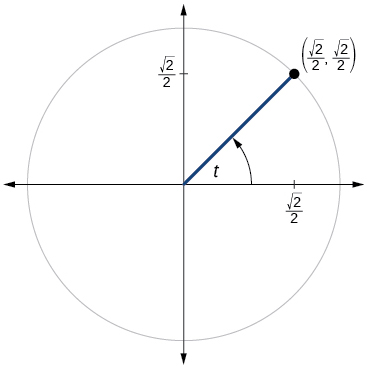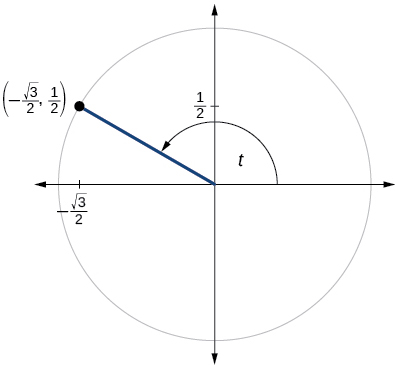$\mathrm{sin}t=\frac{1}{2},\mathrm{cos}t=-\frac{\sqrt{3}}{2}$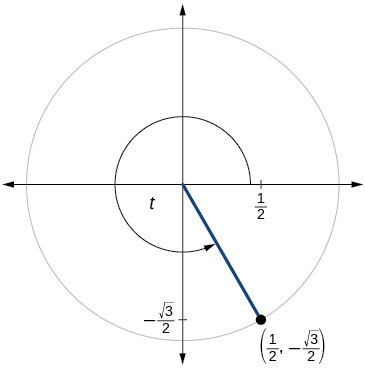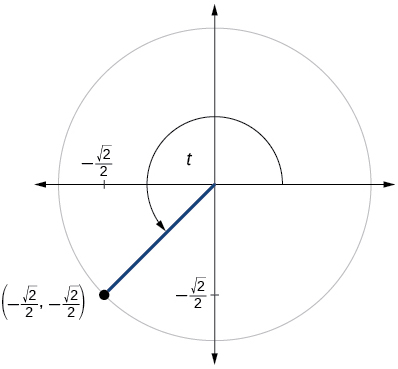$\mathrm{sin}t=-\frac{\sqrt{2}}{2},\mathrm{cos}t=-\frac{\sqrt{2}}{2}$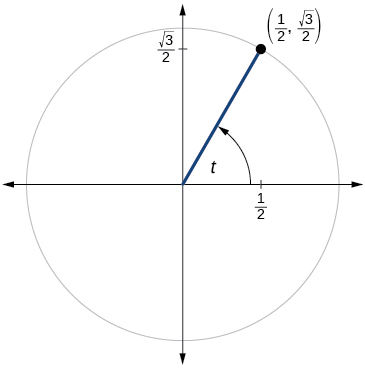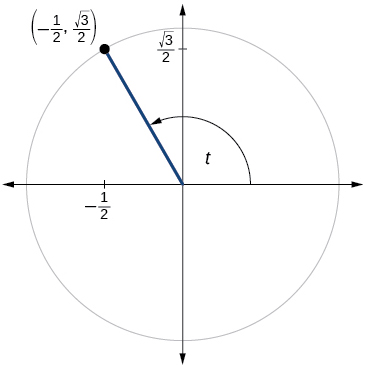$\mathrm{sin}t=\frac{\sqrt{3}}{2},\mathrm{cos}t=-\frac{1}{2}$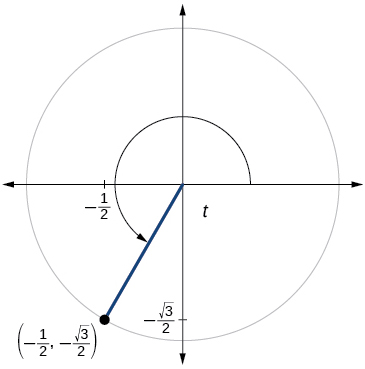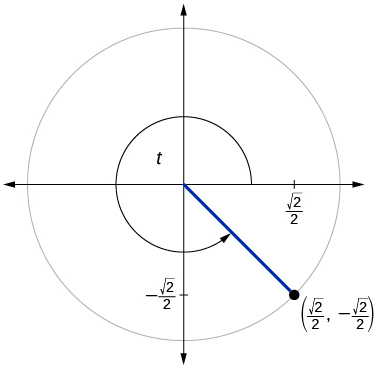$\mathrm{sin}t=-\frac{\sqrt{2}}{2},\mathrm{cos}t=\frac{\sqrt{2}}{2}$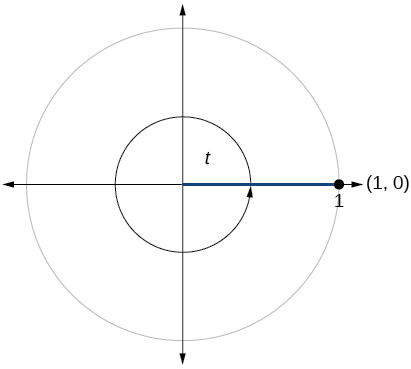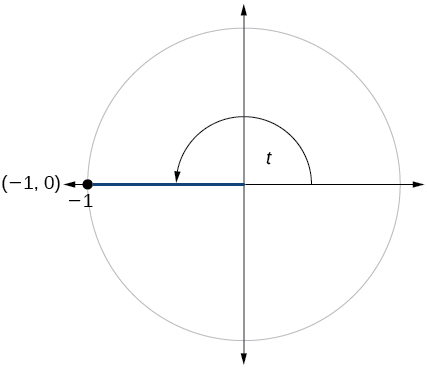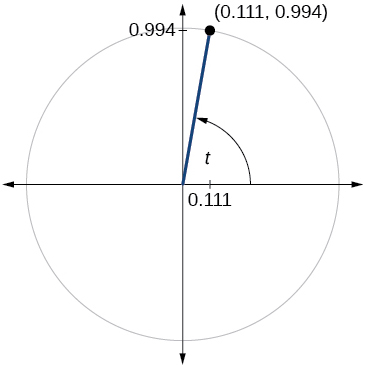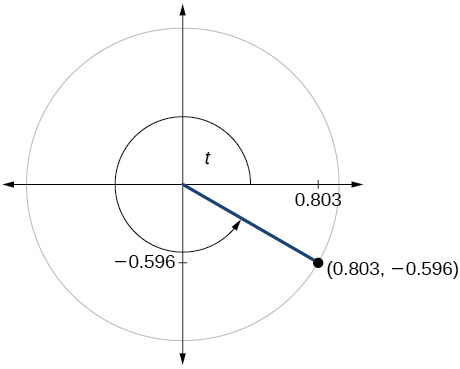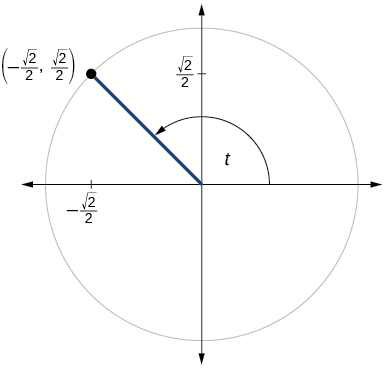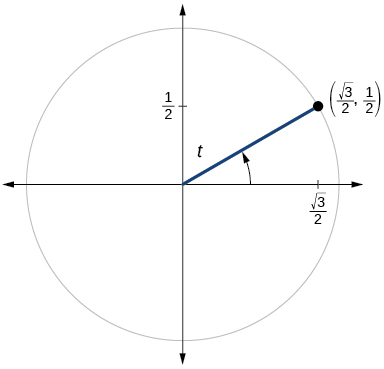$\mathrm{sin}t=\frac{1}{2},\mathrm{cos}t=\frac{\sqrt{3}}{2}$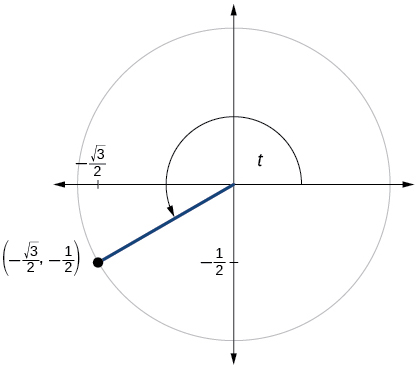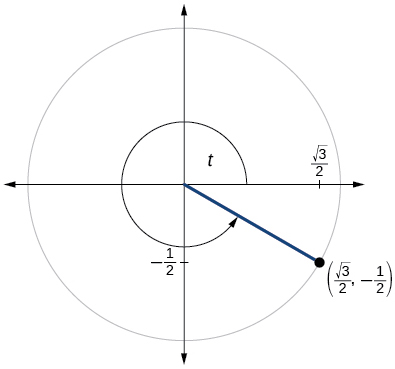$\mathrm{sin}t=-\frac{1}{2},\mathrm{cos}t=\frac{\sqrt{3}}{2}$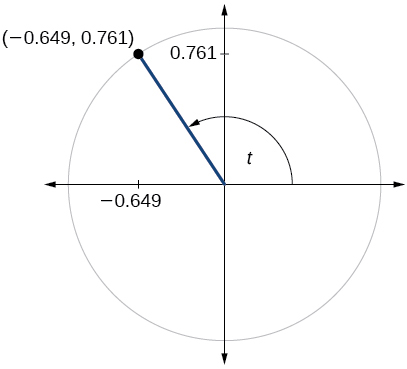$\mathrm{sin}t=0.761,\mathrm{cos}t=-0.649$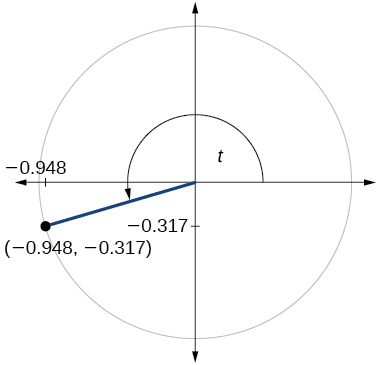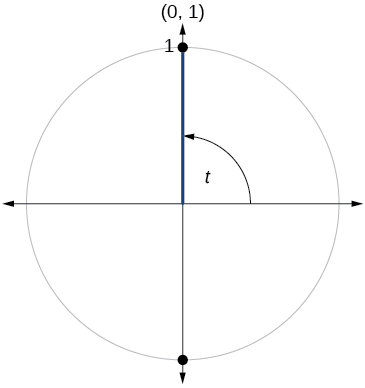$\mathrm{sin}t=1,\mathrm{cos}t=0$

## Technology

For the following exercises, use a graphing calculator to evaluate.

$\mathrm{sin}\text{\hspace{0.17em}}\frac{5\pi }{9}$

$\mathrm{cos}\text{\hspace{0.17em}}\frac{5\pi }{9}$

−0.1736

$\mathrm{sin}\text{\hspace{0.17em}}\frac{\pi }{10}$

$\mathrm{cos}\text{\hspace{0.17em}}\frac{\pi }{10}$

0.9511

$\mathrm{sin}\text{\hspace{0.17em}}\frac{3\pi }{4}$

$\mathrm{cos}\text{\hspace{0.17em}}\frac{3\pi }{4}$

−0.7071

$\mathrm{sin}\text{\hspace{0.17em}}98°$

$\mathrm{cos}\text{\hspace{0.17em}}98°$

−0.1392

$\mathrm{cos}\text{\hspace{0.17em}}310°$

$\mathrm{sin}\text{\hspace{0.17em}}310°$

−0.7660

## Extensions

For the following exercises, evaluate.

$\mathrm{sin}\left(\frac{11\pi }{3}\right)\text{\hspace{0.17em}}\mathrm{cos}\left(\frac{-5\pi }{6}\right)$

$\mathrm{sin}\left(\frac{3\pi }{4}\right)\text{\hspace{0.17em}}\mathrm{cos}\left(\frac{5\pi }{3}\right)$

$\frac{\sqrt{2}}{4}$

$\mathrm{sin}\left(-\frac{4\pi }{3}\right)\text{\hspace{0.17em}}\mathrm{cos}\left(\frac{\pi }{2}\right)$

$\mathrm{sin}\left(\frac{-9\pi }{4}\right)\text{\hspace{0.17em}}\mathrm{cos}\left(\frac{-\pi }{6}\right)$

$-\frac{\sqrt{6}}{4}$

$\mathrm{sin}\left(\frac{\pi }{6}\right)\text{\hspace{0.17em}}\mathrm{cos}\left(\frac{-\pi }{3}\right)$

$\mathrm{sin}\left(\frac{7\pi }{4}\right)\mathrm{cos}\left(\frac{-2\pi }{3}\right)$

$\frac{\sqrt{2}}{4}$

$\mathrm{cos}\left(\frac{5\pi }{6}\right)\text{\hspace{0.17em}}\mathrm{cos}\left(\frac{2\pi }{3}\right)$

$\mathrm{cos}\left(\frac{-\pi }{3}\right)\mathrm{cos}\left(\frac{\pi }{4}\right)$

$\frac{\sqrt{2}}{4}$

$\mathrm{sin}\left(\frac{-5\pi }{4}\right)\text{\hspace{0.17em}}\mathrm{sin}\left(\frac{11\pi }{6}\right)$

$\mathrm{sin}\left(\pi \right)\mathrm{sin}\left(\frac{\pi }{6}\right)$

0

## Real-world applications

For the following exercises, use this scenario: A child enters a carousel that takes one minute to revolve once around. The child enters at the point $\text{\hspace{0.17em}}\left(0,1\right),$ that is, on the due north position. Assume the carousel revolves counter clockwise.

What are the coordinates of the child after 45 seconds?

What are the coordinates of the child after 90 seconds?

$\left(0,–1\right)$

What are the coordinates of the child after 125 seconds?

When will the child have coordinates $\text{\hspace{0.17em}}\left(0.707,–0.707\right)\text{\hspace{0.17em}}$ if the ride lasts 6 minutes? (There are multiple answers.)

37.5 seconds, 97.5 seconds, 157.5 seconds, 217.5 seconds, 277.5 seconds, 337.5 seconds

When will the child have coordinates $\text{\hspace{0.17em}}\left(–0.866,–0.5\right)\text{\hspace{0.17em}}$ if the ride lasts 6 minutes?

#### Questions & Answers

write down the polynomial function with root 1/3,2,-3 with solution
if A and B are subspaces of V prove that (A+B)/B=A/(A-B)
write down the value of each of the following in surd form a)cos(-65°) b)sin(-180°)c)tan(225°)d)tan(135°)
Prove that (sinA/1-cosA - 1-cosA/sinA) (cosA/1-sinA - 1-sinA/cosA) = 4
what is the answer to dividing negative index
In a triangle ABC prove that. (b+c)cosA+(c+a)cosB+(a+b)cisC=a+b+c.
give me the waec 2019 questions
the polar co-ordinate of the point (-1, -1)
prove the identites sin x ( 1+ tan x )+ cos x ( 1+ cot x )= sec x + cosec x
tanh`(x-iy) =A+iB, find A and B
B=Ai-itan(hx-hiy)
Rukmini
what is the addition of 101011 with 101010
If those numbers are binary, it's 1010101. If they are base 10, it's 202021.
Jack
extra power 4 minus 5 x cube + 7 x square minus 5 x + 1 equal to zero
the gradient function of a curve is 2x+4 and the curve passes through point (1,4) find the equation of the curve
1+cos²A/cos²A=2cosec²A-1
test for convergence the series 1+x/2+2!/9x3ByByBy Gerr ZenBy RhodesBy